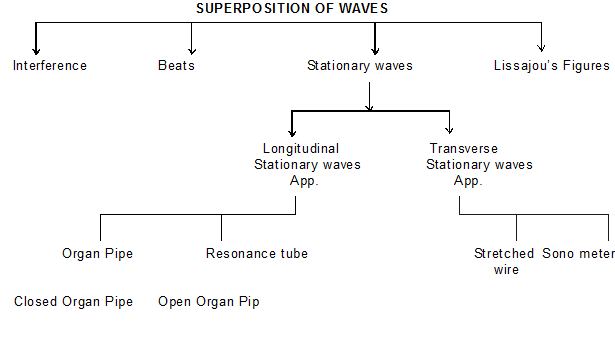Most Affordable JEE | NEET | 8,9,10 Preparation by Kota's Top IITian Doctor Faculties

# Principle of superposition of waves - Types of Interference - eSaralHey do you learn about the Principle of superposition of waves? If so. Then keep reading.

## Principle of Superposition

Two, or more, progressive waves can travel simultaneously in a medium without affecting the motion of one another. Therefore, the resultant displacement of each particle of the medium m any instant is equal to the vector sum of the displacements produced by the two waves separately, this principle is called the 'principle of superposition.

$y=y_{1} \pm y_{2} \pm$

$\vec{y}=\vec{y}_{1}+\vec{y}_{2}+\ldots \ldots \ldots \ldots$

Special Point –
1. It holds for all types of waves, provided the waves are not of very large amplitude. If waves are of very large amplitude, as laser waves, then this principle does not hold.
2. When we listen to an orchestra, we receive a complex sound due to the superposition of sound waves of different characteristics produced by different musical instruments. Still, we can recognize separately the sounds of different instruments.
3. Similarly, our radio antenna is open to the waves of different frequencies transmitted simultaneously by different radio stations. But when we tune the radio of a particular station, we receive the program of that station only as if the other stations were silent. Thus, the principle of superposition holds not only for the mechanical waves but also for the electromagnetic waves.## Interference :

when two waves of the same frequency travel in a medium simultaneously in the same direction then, due to their superposition, the resultant intensity at any point of the medium is different from the sum of intensities of the two waves. At some points, the intensity of the resultant waves is very large while at some other points it is very small or zero. This phenomenon is called the 'interference' of waves.

Mathematically,

Ist wave $\Rightarrow \mathrm{Y}_{1}=\mathrm{a}_{1} \sin (\omega \mathrm{t})$

Ilnd wave $\Rightarrow Y_{2}=a_{2} \sin (\omega t+\phi)$

By principle of Superposition

$y=y_{1}+y_{2}$

$y=a_{1} \sin \omega t+a_{2} \sin (\omega t+\phi)$

$y=a_{1} \sin \omega t+\left[a_{2} \sin \omega t \cos \phi\right.$
$\left.+a_{2} \cos \omega t \sin \phi\right]$

$y=\sin \omega t\left(a_{1}+a_{2} \cos \phi\right)$
$+a_{2} \cos \omega t \sin \phi$

Suppose that

$a_{1}+a_{2} \cos \phi=A \cos \theta$ ……….(1)

$\mathrm{a}_{2} \sin \phi=\quad \mathrm{A} \sin \theta$ ……….(2)

some the square of Eq. (1) & (2)

$A^{2}=a_{1}^{2}+a_{2}^{2}+2 a_{1} a_{2} \cos \phi$

This is amplitude of resultant wave.

Divide Eq. (2) By Eq. (1)

$\tan \theta=\frac{a_{2} \sin \phi}{a_{1}+a_{2} \cos \phi}$ ………………..(3)

$\phi$ Phase difference between two waves

Eq. of Resultant wave

$y=A \sin (w t+\theta)$

$\theta$ Initial phase of resultant wave

For interference I $\propto a^{2}$

$\mathrm{I}=\mathrm{I}_{1}+\mathrm{I}_{2}+2 \sqrt{\mathrm{l}_{1}} \sqrt{\mathrm{I}_{2}} \cos \phi$

### (1) Constructive interference:

When waves are meat in same phase

phase difference

$\Delta \phi \quad=\quad 0,2 \pi, 4 \pi \quad \ldots \ldots 2 n \pi$

Path difference

$\Delta \mathrm{x} \quad=\quad 0, \lambda, 2 \lambda \ldots \ldots . . \mathrm{n} \lambda$

$\mathrm{n} \quad=\quad 0,1,2, \ldots \ldots \ldots \ldots$

$\cos \phi=+1$

$\mathrm{I}_{\max }=\left(\sqrt{I_{1}}+\sqrt{I_{2}}\right)^{2}$

$A_{\max }=a_{1}+a_{2}$

If

$a_{1}=a_{2}=a$

$\mathrm{I}_{1}=\mathrm{I}_{2}=\mathrm{I}$

$\mathrm{I}_{\max }=4 \mathrm{I}$

$A_{\max }=2 a$

### (2) Destructive Interference

Phase difference $\Delta \phi \quad=\quad \pi, 3 \pi \quad \ldots \ldots(2 n+1) \pi$

Path difference $\Delta \mathrm{x} \quad=\quad \frac{\lambda}{2}, \frac{3 \lambda}{2} \quad \ldots \ldots(2 n+1) \frac{\lambda}{2}$

$\begin{array}{lll}n & = & 0,1,2, \ldots \ldots \ldots \ldots \ldots . .\end{array}$

$\mathrm{A}^{2}=\mathrm{a}_{1}{ }^{2}+\mathrm{a}_{2}{ }^{2}+2 \mathrm{a}_{1} \mathrm{a}_{2} \cos \phi$

$\cos \phi=-1$

$\mathrm{I}=\mathrm{I}_{1}+\mathrm{I}_{2}+2 \sqrt{\mathrm{l}_{1}} \quad \sqrt{\mathrm{I}_{2}} \cos \phi$

$\cos \phi=-1$

$A_{\min }=a_{1}-a_{2}$

$\mathrm{I}_{\min }=\left(\sqrt{I_{1}}-\sqrt{I_{2}}\right)^{2}$

If

$a_{1}=a_{2}=a$

$\mathrm{I}_{1}=\mathrm{I}_{2}=\mathrm{I}$

$A_{\min }=0$

$I_{\min }=0$

## Special results about interference pattern

Results
1. Maximum and minimum intensities in any interference wave form. $\frac{\mathrm{I}_{\max }}{\mathrm{I}_{\operatorname{Min}}}=\left(\frac{\sqrt{\mathrm{l}_{1}}+\sqrt{\mathrm{I}_{2}}}{\sqrt{\mathrm{l}_{1}}-\sqrt{\mathrm{I}_{2}}}\right)^{2}=\left(\frac{\mathrm{a}_{1}+\mathrm{a}_{2}}{\mathrm{a}_{1}-\mathrm{a}_{2}}\right)^{2}$
2. Average intensity of interference wave form or $I_{\text {av }}=\frac{\text { I }_{\max }+I_{\min }}{2}$
Put the value of $I_{\max } \& I_{\min }$

Or

$\left.\right|_{\mathrm{av}}=\mathrm{I}_{1}+\mathrm{I}_{2}$

$a=a_{1}=a_{2}$

And

$I_{1}=I_{2}=I$

Then

$I_{\max }=4$I

Then

$I_{\max }=4$I

$I_{\min }=0$

And

$I_{A V}=2 I$

So, that's all from this article. If you liked this article on the principle of superposition of waves then please share it with your friends. If you have any confusion related to this topic then feel free to ask in the comments section down below.

For a better understanding of this chapter, please check the detailed notes of the Waves. To watch Free Learning Videos on physics by Saransh Gupta sir Install the eSaral App.